# 【DB究谈】浅谈对数据库隔离级别的理解

(到底什么是事务隔离级别？为什么要有事务隔离级别？

 时刻 事务 1 事务 2 t0 BEGIN;  // x = 10 BEGIN; t1 w1[x]: 更新  x = x + 1;  // x = 11 t2 r2[x]: read x;  // x = 11，依赖 w1[x] t3 a1: abort(引发回滚 );  // x = 10 t4 更新 y : = x

1.

(1) 按照上述执行序列，事务 2读取的数据是事务 1未提交的脏数据 x = 11，实际上是不应当被事务 2看到的不一致的数据，如果该数据被事务 2后续应用于更新某条记录 y := 11，那么将导致数据库的状态不一致。 (这便是大家所知的 脏读 )

(2) 如果把事务 1放在事务 2之前执行，那么这是串行化的事务执行方式，这时事务 2读到的 x值应该是事务 1未开始前的值 (已回滚 )，即 x = 10，这是正确的结果。具体如下 :

 时刻 事务 1 事务 2 t0 BEGIN;  // x = 10 t1 w1[x]: 更新  x = x + 1;  // x = 11 t2 a1: abort(引发回滚 );  // x = 10，结束 BEGIN;  // x = 10，与事务1串行，无问题 t3 r2[x]: read x;  // x = 10 t4 更新 y : = x ... ...

2.

 -> 并发事务导致执行结果破坏某些约束  (问题源头 ) -> 起因归为读写冲突 (w-w/w-r/r-w) -> 异常现象 (Anomaly)(不完全 )归纳为异常模式 -> 更广义的行为序列 (现象 -Phenomenon)被归纳为行为序列模式 -> 禁止行为序列或异常序列模式可规避某些并发操作问题 -> 根据行为序列模式的归纳来定义 : 解决某些问题即符合特定隔离级别。

(如何标准化、形式化地描述并发读写的 (异常 )现象？如何理解它们？

>>

>> 数据集操作对应于 P3(潜在幻象 )

>> 具有关联约束的数据操作对应于 A5(A5A(r-w-w-r),A5B(r-r-w-w),...)以及其它可能的涉及数据关联约束的行为模式；

 常见现象 异常现象集合 /模式 (原始 ANSI-SQL标准所涉及 ) 普通现象集合 /模式 (包含了相应异常现象， ) (增强版 ANSI-SQL隔离级别 ) e.g. 脏写 记录 w-w 冲突 未定义 A0 P0:  w1[x]...w2[x]...(c1/a1/c2/a2) e.g. 脏读 记录 w-r 冲突 A1:  w1[x]... r2[x]...a1 先写后读 ; P1:  w1[x]...r2[x]...(c1/a1/c2/a2) P1包含 A1; e.g. 不可重复读 记录 r-w 冲突 A2:  r1[x]...w2[x]...c2... r1[x] 先读后写 ; P2:  r1[x]...w2[x]...(c1/a1/c2/a2) P2包含 A2; e.g. 幻象 集合 r-w冲突 A3:  r1[P]...w2[x in P]...c2...r1[P] 先读后写 ; P3:  r1[P]...w2[x in P]...(c1/a1/c2/a2) P3包含 A3; e.g. 写丢失 记录 r-w-w冲突 - P4:  r1[x]... w2[x]...w1[x]...c1 P2包含 P4, 先读后写 ; e.g. 读偏斜 关联数据冲突 A5A:  (属于 A5类模式 ) r1[x]...w2[x]...w2[y]...c2... r1[y] 先读后写 ; P2包含 P4 e.g. 写偏斜 关联数据冲突 A5B:  (属于 A5类模式 ) r1[x]...r2[y]... w1[y]...w2[x]...(c1/c2) 先读后写 ; P2包含 A5 ... (等等 ) ... ...

3.

(1)     行为序列说明 例如， P1: w1[x]...r2[x]...(c1/a1/c2/a2)，要表达的是 事务 1写数据项 x，之后 (还可能经历其它数据项 /事务的操作 )，事务 2写数据 x，之后，事务 1/2以任意顺序和方式 (提交 /中止 )结束；

(2)     行为序列模式的语义 满足某种模式 P的序列可以构成一个集合，集合中有些具体序列 可能产生异常结果，也可能不会。比如，符合 P2模式的序列不一定就符合 A2(脏读 )，而符合 A2的具体序列不一定就会造成真正的影响。 (比如 r2[x]中的读取操作不被任何后续的应用程序或人员的操作锁依赖而仅仅看一下就丢弃了，那么就不会产生影响 )

(3)      A1-A3: 3种异常现象序列模式是 ANSI-SQL定义隔离级别所使用的异常行为序列模式，但是这些描述的是某个具体种类的异常，对记录级读写冲突的描述不够完备，因此论文 中提出了 P0-P3来扩充 A1-A3(比如， A1模式为 : “w1[x]...r2[x]...a1”，是 3元组；而 P1模式为 : “w1[x]...r2[x]...”，是 2元组 (因为任意的结束顺序和方式 (c1/a1/c2/a2)并没有够成对序列模式的限制，因此可忽略 )显然 A1P1的特例 , A2/P2,A3/P3类似 )

(4)      可串行化级别 上述 A1-A3的不完备也说明了基于 A1-A3来定义的 ANSI-SQL隔离级别不够严谨 ( 具体见 ()的表 4 )

(5)      P4: 写丢失现象

a)         P4不同于 P0，虽然都涉及 w-w冲突，但是 P0的模式是 ”w1[x]-w2[x]-...”，而 P4的冲突是 ”r1[x]...w2[x]...w1[x]-...”，相对于 P0在最前面还有一次同一数据项的读取操作，这暗示了后面的 w1[x]有可能依赖于 r1[x]的读取结果，而 P0并没有这一点。

b)        P4”r1[x]...w2[x]...w1[x]-...c1”写丢失现象被包含于 P2” r1[x]...w2[x]...”(先读后写 )

(6)      A5:  具有关联约束的 两个数据项的操作序列，可能会导致关联约束被破坏 (可以是用户自定义的约束 )。需要注意，基于任意多个数据项之间的关联约束并未在文中明确定义，因此在 P0-P3,A5之外还存在着其它的异常现象

a)         A5A 读偏斜 事务 1要读取 2个具有一定约束的数据项，需要 2次读操作 (r1[x]......r1[y])，在它们之间被插入了事务 2的关于写操作 (r1[x]... w1[x]...w2[y]...c2...r1[y]...)，这样在第二次读操作时约束关系已经被破坏了。

b)        A5B 写偏斜 两个事务分别要先读取一个数据项 (r1[x]...r2[y]...)，再根据读到的值来计算并更新另一个数据项 (...w1[y]...w2[x])，如果两个事务无法可串行化的执行，那么可能会出现破坏约束的情况。例如 :初值 x=y=50r1[x=50]... r2[y=50]...w1[y:=x+1=]... ... ... ...

(7)      P2: 不可重复读现象，该现象是比较有意思的 :

a)         P2包含了 A2，这在前面 (3)已经提及。

b)        P2包含了 P4，这在前面 (4)已经提及。

c)         P2包含了 A5AA5B，这在前面已经提及。

(8)     END

Q. 不可重复读现象 (A2/P2)与幻象现象 (A3/P3)的区别与联系是什么？

A. 不可重复读是仅仅针对单条数据。实际上可以认为，幻象是针对数据集合的不可重复读现象，因此幻象不仅包含了 MySQLRR隔离级别所能避免的幻读 (因为插入 数据导致集合不可重复读 )，而是可以扩展到 因为增 //改数据记录而导致的给定谓词条件下的数据集合的不可重复读。

RU(read uncommitted)隔离级别下，通过 WHERE条件相关的数据记录进行增 //改，即 可改变查询结果集。这里需要改变的数据记录是要引起 cond判定的变化的，否则并不能影响查询结果。比如  INSERT一个满足 condkey值， UPDATE一个 key(其旧有或目标 key值满足 cond)DELETE一个 key值，都可以改变满足 cond的查询结果集。

(1) 对于 A1-A2的异常 (A3类似于 A2)可以形象化表示为 V 异常 :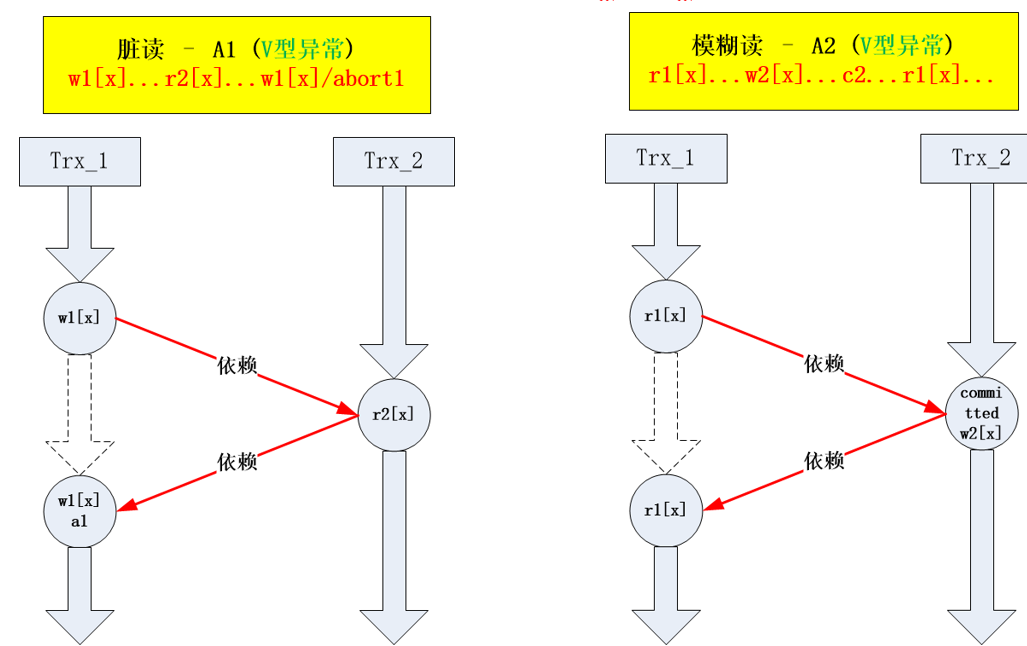1.

(2) 什么是 A5类异常？什么是读倾斜、写偏斜？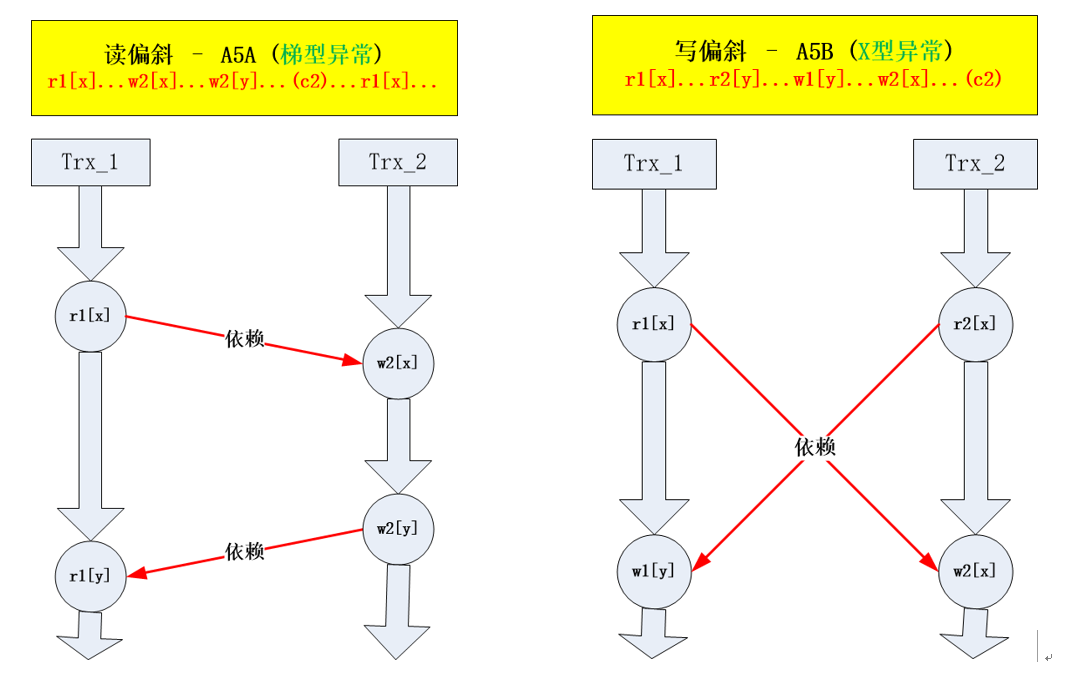2.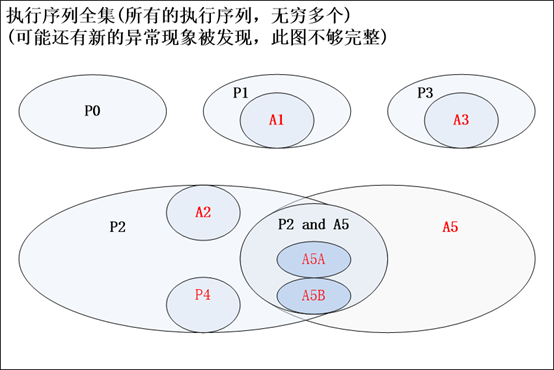3.

(如何严格地定义隔离级别？

1. ANSI-SQL92中定义的 4中隔离级别 (解决 A1-A3中的部分或者全部异常 ):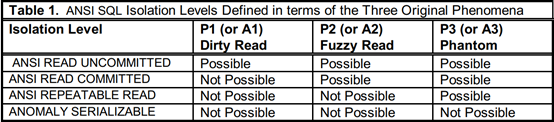4. - -table 1

2. 基于 P0-P3的隔离级别定义 : (来自论文 )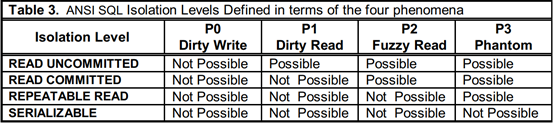5.  - -table 3

3. 基于锁机制的隔离级别定义 : (来自论文 )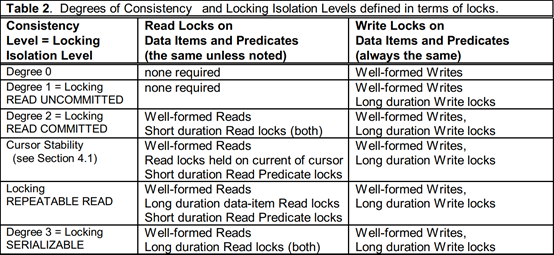6.  - -table 2

 Degree-0 << any defined Isolation Level. ANSI-RU << RU  ==  Locking-RU ==  Degree-1 ANSI-RC << RC  ==  Locking-RC ==  Degree-2 Degree-2 << ANSI-RR << RR  ==  Locking-RR << Degree-3 ANOMALY-SR << SR  ==  Degree-3

4. 最终的隔离级别关系图 (来自论文 )

23的定义 以及  ()中的序列模式关系为基础，最终我们得到了一张隔离级别关系图 :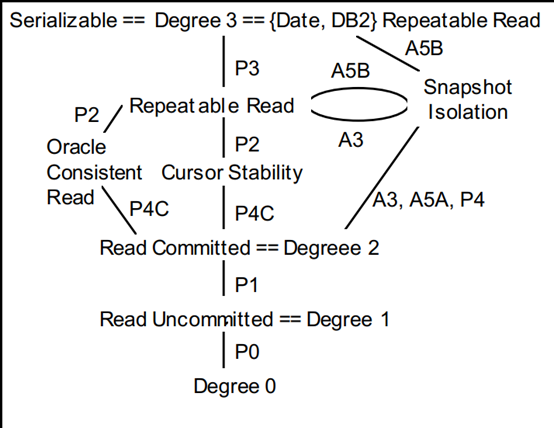4. - -Figure 2

(相关问题

Q.  InnoDB  - RR 隔离级别的意义何在？

A. InnoDB中隔离级别的实现手段有 2种，一种是 ()锁机制，一种是在 MVCC机制 (需要与锁机制结合 )。无论用户发起的事务中的 SQL都是基于锁并发控制的读 /SQL，还是也包含了基于 MVCC(无锁 )的只读 SQL，执行结果都应满足该事务所处隔离级别的语义。

Q. 关于 SI(快照隔离 )级别

A. 论文 中有所描述，是基于 MVCC技术实现的隔离级别，涉及的行为序列属于 多版本 (Multi-Versioning)行为序列，在理论上需要向 单值 (Single-Valued)行为序列做等价转换才能够将 SI并入 4.所示的隔离级别体系结构 (去做比较 )。文中涉及很多相关的分析过程，限于篇幅后续单独再对基于 MVCC技术的隔离级别做讨论。这里仅列举一些重要的结论 ( 4. ):

 Degree-2 == RC << SI << SR = Degree-3 SI >><< RR

Q. P2为何如此包容 (P2包含 A2,P4,A5A,A5B,...)

A. 一方面， P2实际上抽象了 A2,P4,A5A,A5B等异常模式的共性—— 先读后写 ；另一方面， 先读后写 很多时候意味着后写的目标数据对先读出的数据有依赖，这里可能是同一个数据项的依赖，也可能是 2个或者多个数据项的依赖，因此会涉及 A5A,A5B等诸多可能破坏关联约束的现象。

 “A Critique of ANSI SQL Isolation Levels,” Proc. ACM SIGMOD 95, pp. 1-10, San Jose CA, June 1995, © ACM

• 博文量
135
• 访问量
104489